# Most Common Machine Learning Algorithms

Here you will learn about list of machine learning algorithms for beginners.

Hello there everyone! Hope you all are doing well. Today we are among you once again with another article on Machine Learning. We already have covered the introduction part and how to setup your environment for ML, so moving on in this very section we will be discussing on different algorithms that are being used widely in the ML domain. Without investing much of our time here, let’s get started.

## Types of Machine Learning Algorithms

Usually there exist three broader categories of ML algorithms containing several other algorithms within them. Here we will be discussing all of them one by one. Come let us have better insights over them.

Three basic algorithms mentioned above are the Supervised Learning, Unsupervised Learning and Reinforcement Learning. Now let us get a grasp over all three of them one by one.

### 1. Supervised Learning

This is a group of algorithms in which an algorithm consist of a target variable (also called dependent variable) which needs to be predicted form the set of independent variables (predictors). The independent variable that we have in our dataset is used to generate a function that can be used to map the input data to the desired outputs.

The training of the machine to produce the desired output goes on and on until and unless a satisfactory level of output’s efficiency is achieved.

Some examples of Supervised Learning are:

• Regression
• Logistic Regression
• Random Forest
• Decision Tree
• KNN etc.

Note: The independent variables mentioned above are the attributes that you can see in your dataset: the columns, and dependent variable is that column that represents the result (outcome). The training data only contains the independent variables for training the machine and the dependent variable is produced at the later stage.

Here we have provided a dataset for your reference: Dataset.

Though we have also mentioned some sources in our previous article where you can find your desired datasets.

### 2. Unsupervised Learning

This very algorithm approach is different from Supervised approach as the algorithms here do not have and dependent/target variable or we can say these algorithms do not have anything to predict/estimate.

These algorithms are basically used for the clustering purpose of the population in different groups.

Some examples of Unsupervised Learning are:

• K-means
• Apriori Algorithm

### 3. Reinforcement Learning

As we have seen and what our motive is in ML, this algorithm also do entertain the basic requirement that the machine is trained to perform specific tasks. The difference here is: machine is exposed to an environment where it learns from past experiences and tries to grasp the best possible way for efficient decision results. This approach involves trial and error.

Some examples of Reinforcement Learning are:

• Markov Decision Process

Now as we have discussed the three basic types of algorithms that rule the ML domain, we now need to get some deeper insights over them. So, we are expected to explain all the algorithms that we have mentioned as an example in each of the learning processes and some more very crucial algorithms that you gonna need as a data scientist.

## Important Machine Learning Algorithms

Here we are going to explain those algorithms that play special roles in the data science and analytics world. So let’s get started quickly.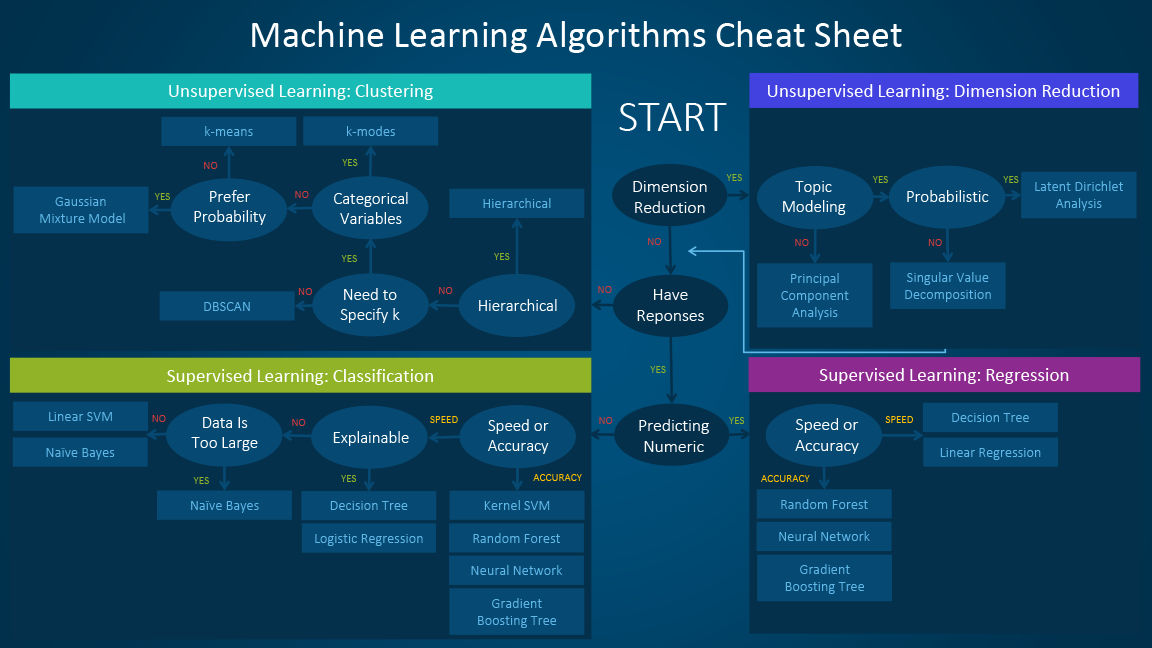Image Source

How to use this Cheat Sheet?

It is quite simple and easy to understand it’s working and make use of it. Let’s understand how:

Suppose you want to do Probabilistic Dimensionality Reduction then according to the sheet you should go for Latent Dirichlet Analysis.

Isn’t that simple? I’m pretty sure you guys will now be convenient with the use of this sheet, if not please let us know in the comments section, we are here always to help you out.

Now let’s very quickly jump to the Algorithms without any further delay:

### 1. Linear Regression

Being the simplest among other algorithms LR (Linear Regression) is primarily used for the estimation/prediction of real values. For example: estimating the cost of a house, total sales, etc.

In LR the relationship is established between dependent and independent variables with the help of a line called as Best Fit line or Regression line.

Best Fit line is represented by a linear equation:  Y = m*X + c.

Where  Y: Dependent Variable

m: Slope

X: Independent Variable

c: Intercept

The coefficients ‘m’ and ‘c’ are obtained by minimizing the sum of squared difference of the distance between the data points and the best fit line.

Let us understand this with the help of an example:

Suppose we are having the best fit line with linear equation: Y = 0.28X + 13.9

According to the problem statement we have to find the weight of a person if height is known. And our motive is achieved using the Linear Regression as shown below: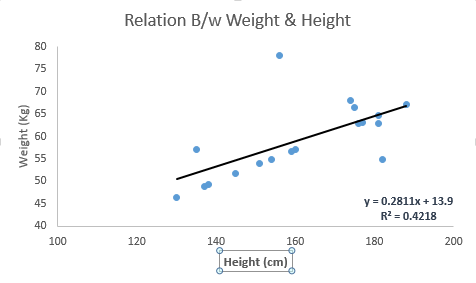Image Source

Linear Regression again is sub-divided into two parts-

Simple LR: having single independent variable

Multiple LR: having more than one independent variable

### 2. Logistic Regression

Despite of having ‘regression’ in the name, it is a classification algorithm and is primarily used for estimation of the discrete values i.e. 0/1, true/false, etc. from the set of available independent variables.

The main aim of this algorithm is to predict the possibility of occurrence of an event and to achieve this, a function is used called Logit Function. Hence this technique is also being known by the name Logit Regression.

The visualization in case of Logit Regression will look something like this: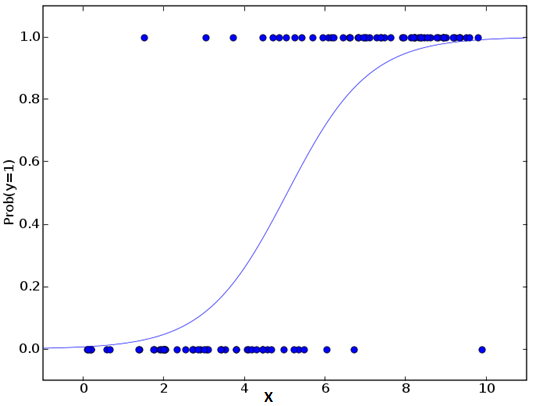Image Source

Some of the steps that are followed by the data scientists to improve the model are:

• Including interaction terms
• Regularization
• Using non-linear model
• Feature removal

### 3. Support Vector Machine (SVM)

This algorithm is based on classification approach. In this the data points are plotted as a point in n-dimensional space, where ‘n’ is the number of features in our dataset. The value of each feature is the value of the particular coordinate.

Let’s understand this too with an example: Suppose initially we have two features- Hair length and Height (also known as Support Vectors). Since we have only two variables, we would plot them in a two-dimensional space and each of the point will be having two coordinates according to the convention.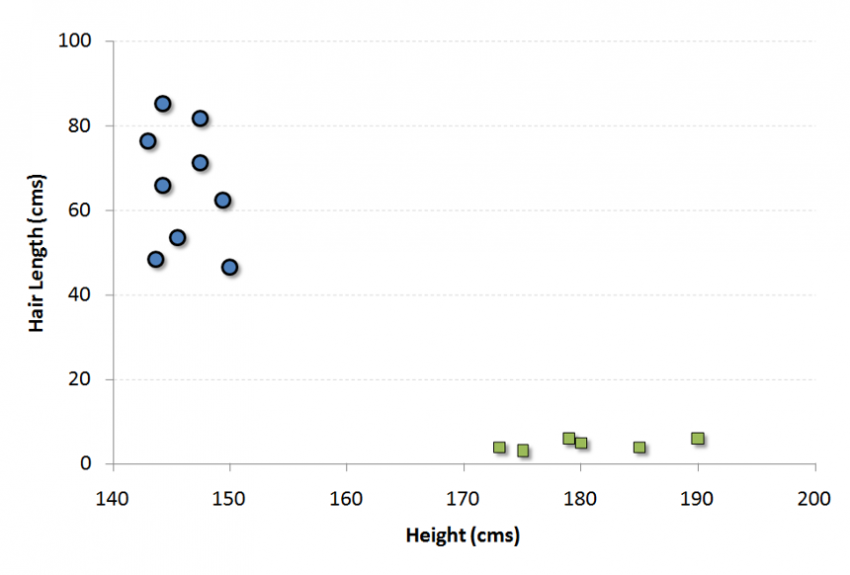Image Source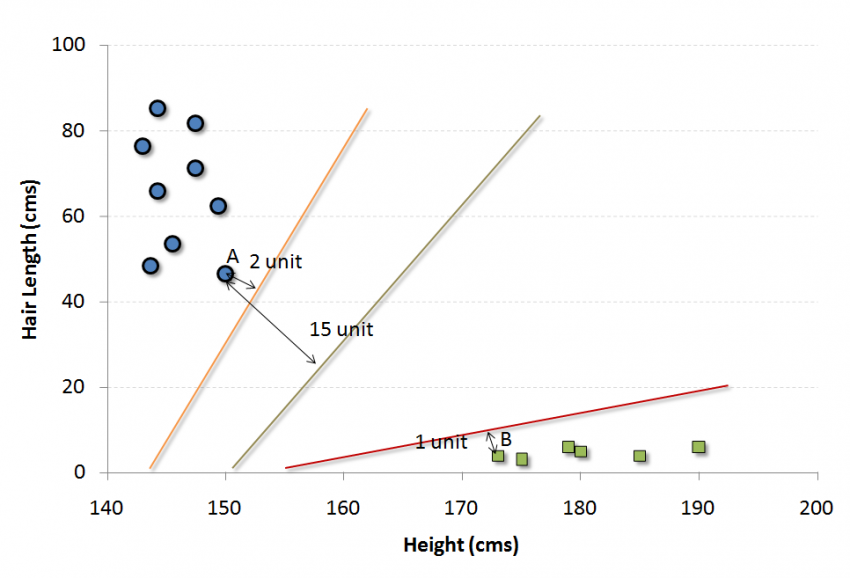Image Source

The black line in the figure above is splitting the data in to two different groups and as we can see that the two closest points are the one farthest from the line, therefore this line is our Classifier. And the new data is classified solely based in the fact that on which side of the line do testing data lands.

### 4. K-Nearest Neighbors (KNN)

KNN approach ignites dual behaviour i.e. can be used for both regression and classification. On the other hand it is observed and suggested by the data scientists for classification problems. This algorithm has a simple action plan- It accepts all the available cases and classify these cases on the basis of majority of votes from its ‘k’ neighbours. So, the case which is being decided for the class is decided in such a way that it must be common to the k neighbours when measured with the help of distance function.

Now let us make this simple to understand by mapping it to our daily lives example: Suppose you want to know about a person you’ve never met before and completely unaware of, then your motive can be fulfilled by enquiring about him/her from the person he/she is in contact with (relate this with k-neighbours). You can take reference from the image below.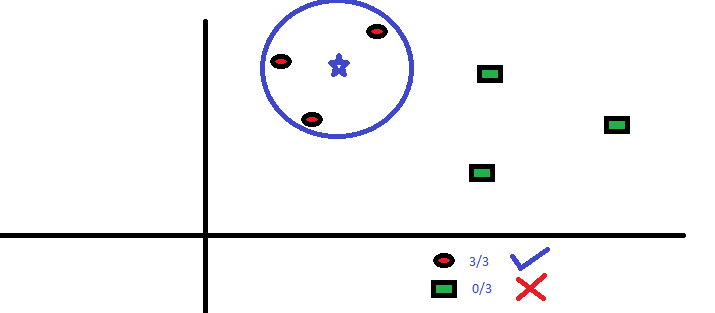Image Source

Dear readers before deciding to opt for K-NN consider these points for better approachability.

• The variables used are required to normalized in order to restrain it from being biased by other variables.
• It is more oriented towards noise removal, removing outliers, etc. rather than actual work.
• K-NN is comparatively expensive than other algorithms.

### 5. Decision Trees

As theme of this algorithm can be inferred from its name itself that resemble to the trees in data structures. The very similar approach is carried out here too.

Decision Tree algorithm to solve the problems is a descendent of Supervised Learning and is one of the favourite among the data scientists and engineer. Though this can be used for both classification and regression but is famous for classification more. In this approach the population is splitted in two or more possible distinct homogeneous sets strictly based on the independent variables.Image Source

You can clearly spot in the image above how the population is being splitted based on different independent variables (attributes) to identify ‘if they will play or not.’ Decision tree uses different techniques like chi-square, Information Gain for splitting the population into various groups, to have detailed information on these techniques do refer to this webpage.

Bonus Tip:  to grasp a vivid knowledge on the Decision Trees do refer to a website famous for machine learning and data sciences: Analytics Vidya.

### 6. Random Forest

Random Forest is the broader term used in reference to the decision trees. It is named as Random Forest because of the fact that it is a collection of decision trees. It comes under the Supervised Learning and can be used for the classification as well as regression.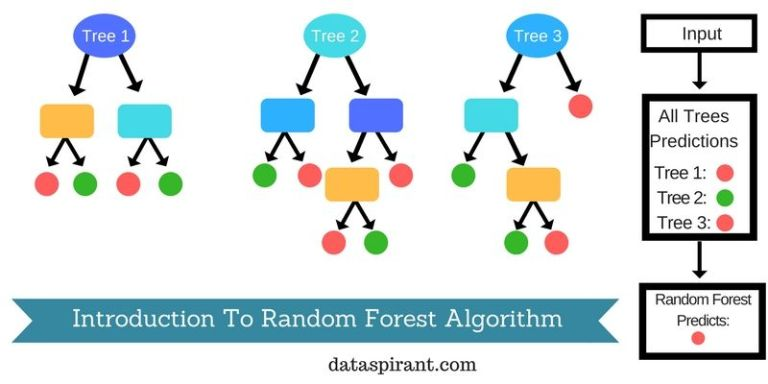Image Source

For the classification of the new object on the basis of independent variables (attributes), vote is casted from each of the tree for the election of the classification. And the classification with maximum votes from the trees is chosen as visible in the image above.

So readers, these were the some (not all) of the most famous machine learning algorithms that we have covered here. In our later blogposts we will be covering Gradient Boosting Algorithms and more. We hope you  guys are having a great learning experience with us.

If there are any queries or suggestions please let us know in the comment section below. Till then have a good day.